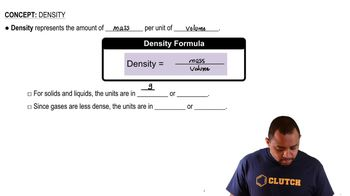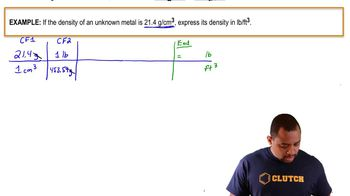Start typing, then use the up and down arrows to select an option from the list.
1:53 minutes
Problem 8
Textbook Question

# Consider 20 mL samples of the following liquids. Which sample has the largest mass? (LO 1.9) (a) Water (density = 1.0 g/mL) (b) Glycerol (density = 1.26 g/mL) (c) Ethanol (density = 0.79 g/mL) (d) Acetic acid (density = 1.05 g/mLVerified Solution
This video solution was recommended by our tutors as helpful for the problem above.
103views